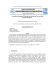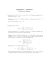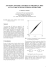# Derivative of the Inverse of ...

## Transcription

Derivative of the Inverse of ...
```Derivative of the Inverse of a Function
One very important application of implicit diﬀerentiation is to ﬁnding deriva­
tives of inverse functions.
√
We start with a simple example. We might simplify the equation y = x
2
(x > 0) by squaring both sides
√ to get y = x. We2 could use function notation
here to say that y = f (x) = x and x = g(y) = y .
In general, we look for functions y = f (x) and g(y) = x for which g(f (x)) =
x. If this is the case, then g is the inverse of f (we write g = f −1 ) and f is the
inverse of g (we write f = g −1 ).
How are the √
graphs of a function and its inverse related? We start by
graphing f (x) = x. Next we want to graph the inverse of f , which is g(y) = x.
But this is exactly the graph we just drew. To compare the graphs of the
functions f and f −1√we have to exchange x and y in the equation for f −1 . So
to compare f (x) = x to its inverse we replace y’s by x’s and graph g(x) = x2 .
f −1 (x) = x2
y=x
f (x) =
√
x
Figure 1: The graph of f −1 is the reﬂection of the graph of f across the line
y=x
In general, if you have the graph of a function f you can ﬁnd the graph of
f −1 by exchanging the x- and y-coordinates of all the points on the graph. In
other words, the graph of f −1 is the reﬂection of the graph of f across the line
y = x.
dy
This suggests that if dx
is the slope of a line tangent to the graph of f , then
dx
1
= dy
dy
dx
1
is the slope of a line tangent to the graph of f −1 . We could use the deﬁnition
of the derivative and properties of inverse functions to turn this suggestion into
a proof, but it’s easier to prove using implicit diﬀerentiation.
Let’s use implicit diﬀerentiation to ﬁnd the derivative of the inverse function:
y
f −1 (y)
d −1
(f (y))
dx
=
f (x)
=
x
d
(x) = 1
dx
=
By the chain rule:
d −1
dy
(f (y))
= 1
dy
dx
so
d −1
1
(f (y)) =
.
dy
dy
dx
Implicit diﬀerentiation allows us to ﬁnd the derivative of the inverse function
x = f −1 (y) whenever we know the derivative of the original function y = f (x).
2
MIT OpenCourseWare
http://ocw.mit.edu
18.01SC Single Variable Calculus��
Fall 2010 ��
```

### Honors HW – Direct and Inverse Variation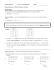### Terrific Tuesday!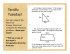### Recent Theory and Applications on Inverse Problems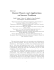### Helping Your Family Save Money | Kids.gov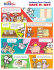### Frederic Weidling Thorsten Hohage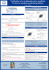### Math 220 Calculus I Exam 2 Fall 13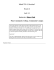### Inverse Functions Activity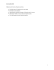### Worksheet 4.8 Composite and Inverse Functions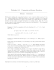### EXAMPLES To divide by a fraction, multiply by its multiplicative inverse... . 5 .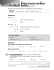### SERGIOS AGAPIOU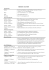### FortiGate firewall Quick Integration Guide for PacketFence version 4.5.0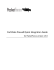### Removing a green screen background in Photoshop 1.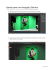### Rearranging Equations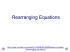### Inverse Functions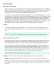### #CHALLENGEYOURSELF Get on your bike and cycle to work with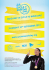### Referential hierarchies: A new look at some historical and typological...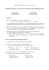### Subduction history of the Farallon plate under North America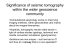### The algebraic theory of partial symmetries (or why groups aren’t enough)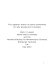### Conference "SPECTRAL AND ANALYTIC INVERSE PROBLEMS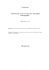### Levenberg-Marquardt Method for Solving the Inverse Heat Transfer Problems